Students can download Maths Chapter 3 Algebra Ex 3.4 Questions and Answers, Notes, Samacheer Kalvi 10th Maths Guide Pdf helps you to revise the complete Tamilnadu State Board New Syllabus, helps students complete homework assignments and to score high marks in board exams.

## Tamilnadu Samacheer Kalvi 10th Maths Solutions Chapter 3 Algebra Ex 3.4

Question 1.
Reduce each of the following rational expression to its lowest form.
(i) $$\frac{x^{2}-1}{x^{2}+x}$$
$$\frac{x^{2}-1}{x^{2}+x}$$ = $$\frac{(x+1)(x-1)}{x(x+1)}$$ = $$\frac { x-1 }{ x }$$

(ii) $$\frac{x^{2}-11 x+18}{x^{2}-4 x+4}$$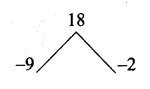x2 – 11x + 18 = (x – 9) (x – 2)
x2 – 4x + 4 = (x – 2) (x – 2)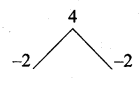(iii) $$\frac{9 x^{2}+81 x}{x^{3}+8 x^{2}-9 x}$$
9x2 + 81x = 9x(x + 9)
x3 + 8x2 – 9x = x(x2 + 8x – 9)
= x (x + 9) (x – 1)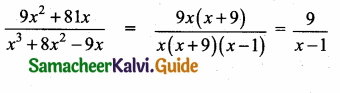(iv) $$\frac{p^{2}-3 p-40}{2 p^{3}-24 p^{2}+64 p}$$
p2 – 3p – 40 = (p – 8) (p + 5)
2p3 – 24p2 + 64p = 2p (p2 – 12p + 32)
= 2p (p – 8) (p – 4)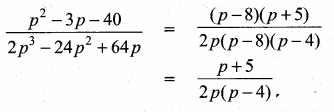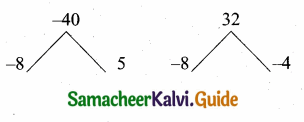Question 2.
Find the excluded values , if any of the following expressions.

(i) $$\frac{y}{y^{2}-25}$$
The expression $$\frac{y}{y^{2}-25}$$ is undefined
when y2 – 25 = 0
y2 – 52 = 0
(y + 5) (y – 5) = 0
y + 5 = 0 or y – 5 = 0
∵ y = -5 or y = 5
The excluded values are -5 and 5(ii) $$\frac{t}{t^{2}-5 t+6}$$
The expression $$\frac{t}{t^{2}-5 t+6}$$ is undefined
when t2 – 5t + 6 = 0
(t – 3) (t – 2) = 0t – 3 = 0 or t – 2 = 0
t = 3 or t = 2
The excluded values are 2 and 3

(iii) $$\frac{x^{2}+6 x+8}{x^{2}+x-2}$$
x2 + 6x + 8 = (x + 4) (x + 2)
x2 + x – 2 = (x + 2) (x – 1)The expression $$\frac { x+4 }{ x-1 }$$ is undefined
when x – 1 = 0
∵ x = 1
The excluded value is 1(iv) $$\frac{x^{3}-27}{x^{3}+x^{2}-6 x}$$
x3 – 27 = x3 – 33
= (x – 3) (x2 + x + 3)
x3 + x2 – 6x = x(x2 + x – 6) = x (x + 3) (x – 2)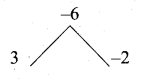when x (x + 3) (x – 2) = 0
x = 0 or x + 3 = 0 or x – 2 = 0
x = 0 or x = -3 or x = 2
The excluded values are 0 , -3 and 2